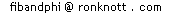# Why do the Fibonacci numbers appear as the number of rabbits in the field each month?

If we let f(n) mean "the number of pairs of rabbits in the field at the start of the n-th month", we will show that f(1)=1, f(2)=1 and f(n)=f(n-1)+f(n-2) which is exactly the definition of the Fibonacci numbers (which also have f(0)=0).

First, we start month 1 with one newly born pair, so

f(1)=1

There is also 1 pair only during month 2 since they are not mature enough to have "babies" yet, so

f(2)=1

Since we assume they mate at age 2 months then a new pair is born at the start of month 3. So, after the second month, how many rabbits will there be? i.e. What is f(n)?
All the rabbits from the previous month (there will be f(n-1) of them) survive, so there are at least f(n-1) of them. How many new ones are born? Any rabbit (pair) that was alive 2 months ago is now able to produce a new pair, and we are assuming they always will and each produces only 1 new pair per month. Thus the number of newly born pairs is the same as the number of pairs 2 alive 2 months ago: f(n-2). Since all the rabbit were alive last month OR are newly born this month, we have:

f(n)=f(n-1)+f(n-2) if n>2
which is just the definition of the Fibonacci numbers (starting with 0 and 1).© 1996-2006 Dr Ron Knottupdatedupdated 1 October 1999